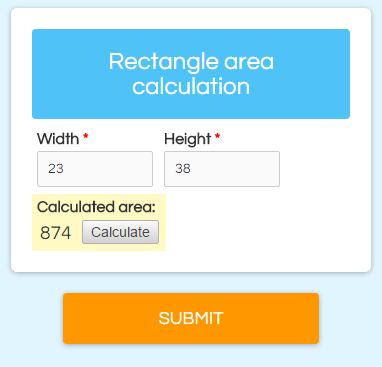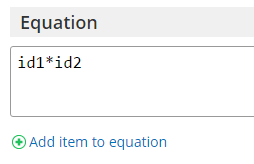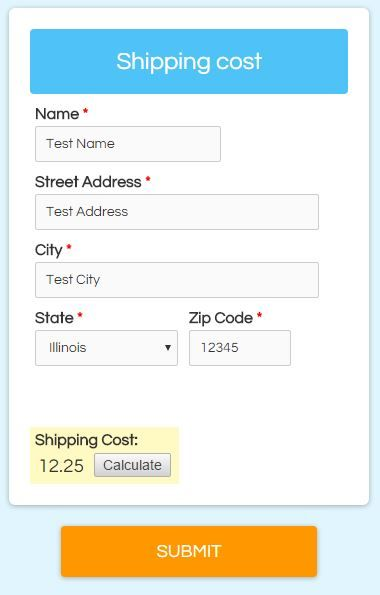# Calculations for Order Forms & Custom CalculatorsOne of the many features that makes Formsite popular and powerful is the Calculations item. This item lets form builders perform basic mathematical equations on form answers, then uses the equation result later in the form.

Our Order Form items make building order forms simple and the Calculations item isn’t needed in those cases. If the simple order form items aren’t enough, however, the Calculations item lets you specify a custom equation.

Some uses for calculations include:

• Order additions (tax, shipping, etc.)
• Scoring calculations
• Custom calculators

The basic use is to first add your items to calculate, then add the Calculation item where you want it to appear. It will ask you for the equation when you add the item, so you can use the Add item to equation link to see all of your qualifying items.

## Making a Calculator

An easy place to start is to make a calculator to determine the area of a rectangle.

1. Add a Number item labeled ‘width’.
2. Add a Number item labeled ‘height’.
3. Add a Calculation item labeled ‘Calculated area:’.

The equation is width * height. Similar to piping, calculation items have their own id numbers that we insert into the equation:

1.Click Add item to equation
2. Locate the item you want to add to the equation
3. Copy the code and click the Close button
4. Paste or enter the code into the correct place in the equation

If the ‘width’ item has a code of ‘id23’ and the ‘height’ item has a code of ‘id24’, the equation would look like:
`id23*id24`

See it in action ›

##Use Scoring to assign values

Calculating basic numbers is easy, but what if you wanted to assign a value to a text option? For example, to set the shipping cost for your order form based on the shipping address State field:

2. Click the Calculations tab
3. Click the box to Enable Scoring
4. Set the Assign values for: setting to Each choice
5. Set your shipping costs for the states
6. Click Save
7. Add a Calculation item labeled ‘Shipping Cost’ with the equation as simply the id number for the State item
8. Check the box next to Include in Order Total
9. Save and test

See it in action ›

## Complex Calculations

You can create virtually any calculation as long as the equation uses the basic mathematical operators +-*/. You can group statements using parentheses and insert constants as regular numbers.

Examples:

1. The order form has 6 products and I want to calculate the total ordered and add a 6% tax:
`(id1+id2+id3+id4+id5+id6)*1.06`
2. The quiz has 5 questions (with values set to 1 for the correct answers) and I want to display the percentage correct:
`((id1+id2+id3+id4+id5)/5)*100`
Note that you can set the Calculation item to show 0 decimal points and provide the ‘%’ suffix to show the percentage as a formatted score.
3. Automatically apply a discount based on the order total. See where we’ve covered this before.